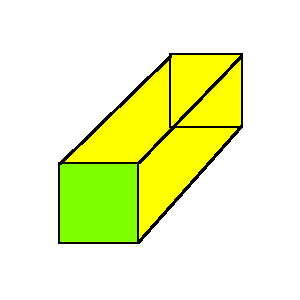The base of the rectangular prism is in the form of a ________.

# The base of the rectangular prism is in the form of a ________.1. A
Square
2. B
Rectangle
3. C
Circle
4. D
Triangle

Fill Out the Form for Expert Academic Guidance!l

+91

Live ClassesBooksTest SeriesSelf Learning

Verify OTP Code (required)

### Solution:

All the faces of the rectangular prism are rectangles. The faces that are opposites are equal. The rectangular prism is also known as a cuboid. There is a shape made on the base of a polyhedron. The base of the rectangular prism is in the form of a Rectangle
Hence, Option (2) is the correct Ans:.

## Related content

 Area of Square Area of Isosceles Triangle Pythagoras Theorem Triangle Formula Perimeter of Triangle Formula Area Formulae Volume of Cone Formula Matrices and Determinants_mathematics Critical Points Solved Examples Type of relations_mathematics+91

Live ClassesBooksTest SeriesSelf Learning

Verify OTP Code (required)